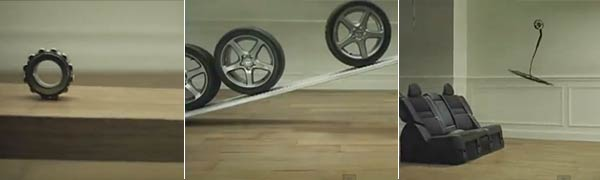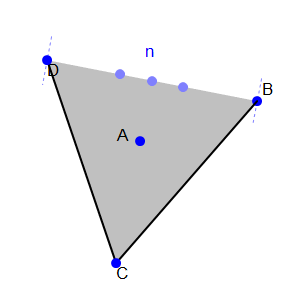Probability

# Expected Value: Level 3 ChallengesIn this car advertisement by Honda, the various parts of the car are arranged to perform physical tricks, which is done sequentially in 60 second shot. The final cut of "Cog" consists of two continuous sixty-second dolly shots taken from a technocrane, stitched together later in post-production. It took 606 takes to make this video!

Each of the interactions we see, from carefully balanced see-saws to swinging mobiles of glass windows, has a certain probability of succeeding each time it is run. If each interaction takes the same amount of time, and we wanted to minimize the total amount of time taken to produce this video, how should the stunts be arranged?

###### Image credit: HondaAn infinite line of stepping stones stretches out into an infinitely large lake.

A frog starts on the second stone.

Every second he takes a jump to a neighboring stone. He has a 50% chance of jumping one stone closer to the shore and a 50% chance of jumping one stone further away from the shore.

What is the expected value for the number of jumps he will take before reaching the first stone (the one closest to the shore)?

###### Other Expected Value QuizzesSammy is lost and starts to wander aimlessly. Each minute, he walks one meter forward with probability $\frac{1}{2}$, stays where he is with probability $\frac{1}{3}$, and walks one meter backward with probability $\frac{1}{6}$.

After one hour, what is the expected value for the forward distance (in meters) that Sammy has traveled?Consider the $N$ vertices of a regular $N-$gon that is inscribed in a unit circle. There are ${ N \choose 3 }$ possible subsets of 3 vertices, which form triangles. If the expected area of the triangle is

$\frac{ 5 \sqrt{ 5 + 2 \sqrt{5} } } { 24 } ,$

what is the value of $N$?

An inventor has made a fantastic new machine that can do many household chores. But what the inventor doesn't know is that he accidentally coded the machine to have a $\frac{5}{12}$ chance of dusting the house, $\frac{1}{3}$ chance of watering the garden and a $\frac{1}{4}$ chance of cleaning the dishes. Every five minutes, the robot switches to do something new. So, by the end of one hour, how many times can we expect that the invention is going to clean the dishes?

×